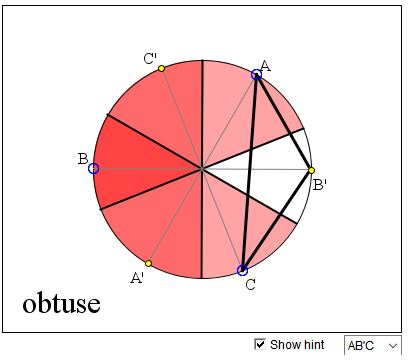## Eight Selections in Six Sectors What is this about? A Mathematical Droodle

### If you are reading this, your browser is not set to run Java applets. Try IE11 or Safari and declare the site https://www.cut-the-knot.org as trusted in the Java setup.What if applet does not run?

ExplanationThe applet attempts to suggest an argument in favor of the thesis that there are 3 times as many obtuse triangles as there are acute ones.

Three points A, B, C are distributed uniformly on a circle. What is the probability of their forming an obtuse triangle?N. Vasiliev credits V. V. Fok and Yu. V. Chekanov with the following argument.

We shall select a triangle in two steps. First we get three points A, B, C and their antipodes A', B', C' across the center of the circle. Selecting a triangle consists in selecting one of the points in each of three pairs {A, A'}, {B, B'}, {C, C'}. There are 8 possible combinations.

With each of the six points we associate the semicircle around its antipode. Three points form an obtuse triangle if and only if they lie in a semicircle and this is only if their associated semicircles have a nonempty intersection. The diameters of the associated semicircles form 6 sectors. Each sector serves as an intersection of the associated semicircles for exactly one selection of the vertices, meaning that out of 8 possible selections there are always 6 obtuse (and 2 acute) triangles. The probability that one is obtuse is therefore,

6/8 = 3/4.

### References

1. N. Vasiliev, Geometric Probability, Kvant (Russian), #1, 1991, 48-53### Geometric Probability